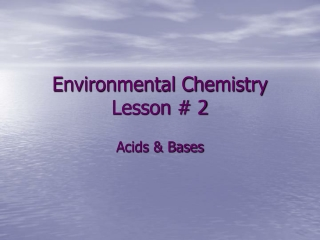DownloadDownload PresentationEnvironmental Chemistry Lesson # 2

# Environmental Chemistry Lesson # 2

Download Presentation## Environmental Chemistry Lesson # 2

- - - - - - - - - - - - - - - - - - - - - - - - - - - E N D - - - - - - - - - - - - - - - - - - - - - - - - - - -
##### Presentation Transcript

1. Environmental Chemistry Lesson # 2 Acids & Bases

2. Properties of Acids & Bases • Acids and bases are water soluble compounds that share some properties with ionic compounds (like sodium chloride) and molecular compounds (like hydrocarbons).

3. Ionization Sulfuric Acid in Water: Aluminum Hydroxide in Water:

4. Strong and Weak Acids • Most acids are highly soluble in water, and the more soluble they are, the more they can conduct electricity. This is due to ionization. • Hydrochloric acid is a better conductor of electricity than acetic acid because every HCl molecule completely dissolves (ionizes) in water to form hydrogen and chlorine ions. In acetic acid, only just over 1% ionizes. • Because of the difference in ionization, hydrochloric acid is considered a strong acid, and acetic acid to be a weak acid. The same holds true with bases.

5. Concentration vs. Strength • The concentration of a substance depends on the amount of solute dissolved in the solvent. • A dilute solution has a relatively small amount of solute per unit volume of solution. • A concentrated solution has a relatively large amount of solute per unit volume of solution. • Both that strong and weak acids can be concentrated or dilute – there is a difference between the amount of solute and the dissociation/ionization of the solute.

6. pH • The pH of a solution is the concentration of hydrogen ions in a solution. • The pH scale runs from 0 to 14 at room temperature, with acids have a relatively low pH, bases have a high pH, and water having a neutral pH in the middle of the scale around 7. • pH is expressed as the negative of the exponent to the base 10 of the hydrogen ion concentration. • For example, a solution with a pH of 3 would have a concentration of 10-3 mol/L. A solution with a pH of 8 would have a concentration of 10-8 mol/L. • pH = -log [H+] • [H+] = 10 -pH

7. Example 1 What is the pH of a solution with a concentration of 2.5 x 10-4 mol/L?

8. Example 2 What is the pH of a solution with a concentration of 9.3 x 10-11 mol/L?

9. Example 3 What is the concentration of a solution with a pH of 3.7?

10. Example 4 • What is the concentration of a solution with a pH of 12.5?

11. Acid-Base Neutralization • When a strong acid and strong base are mixed, a neutralization reaction occurs. • Neutralization is a double displacement reaction, where the products are always water, and a soluble salt. • The hydrogen from the acid and the hydroxide from the water combine to form water. • If the acids or bases are weak, a complete neutralization does not occur.

12. Example 5 Sulfuric Acid + Sodium Hydroxide →

13. Example 6 Aluminum Hydroxide + Phosphoric Acid →

14. Acid-Base Titration • A titration is used to determine the concentration of a substance in solution. It is a method of quantitative chemical analysis. • A known volume of the sample to be analyzed (either an acid or a base) is put into an Erlenmeyer flask. • A buret contains a solution of an accurately known concentration, called a standard solution, or titrant. If you are analyzing an acid, then a base would be in the burette, and vice versa. • Alternatively, the known solution can be in the flask, and the unknown can be in the buret. • The titrant is added drop by drop until the reaction between the two chemicals is judged to be complete – this is usually determined by a colour change. This is called the endpoint. • At least three trials of titration should be doing to accurately determine the concentration of the unknown solution. • Knowing the mole ratios from the balanced equation, and the amounts (in L) of each substance used determines the concentration of the unknown solution.

15. Titration Setup

16. Example 7 A 10.0 mL sample of hydrochloric acid is titrated with a standardized 0.2 mol/L solution of sodium hydroxide. 13.05 mL of sodium hydroxide was used to neutralize the acid. What is the concentration of the hydrochloric acid?

17. Example 8 A 10.0 mL standard solution of 0.150 mol/L nitric acid is used to determine the concentration of sodium carbonate. 12.80 mL of sodium carbonate was used in the titration. What is the concentration of sodium carbonate?